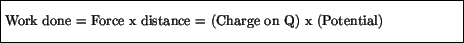Next: Current Up: Charges in motion Previous: Charges in motion

## Electric potential

If the electrical force moves a charge a certain distance, it does work on that charge. The change in electric potential over this distance is defined through the work done by this force:where potential is shorthand for change in electric potential, or potential difference. This is analogous to the definition of the gravitational potential energy through the work done by the force of gravity in moving a mass through a certain distance. The units of potential difference, or simply potential, are Joules / Coulomb, which are called Volts (V). Physically, potential difference has to do with how much work the electric field does in moving a charge from one place to another. Batteries, for example, are rated by the potential difference across their terminals. In a nine volt battery the potential difference between the positive and negative terminals is precisely nine volts. On the other hand the potential difference across the power outlet in the wall of your home is 110 volts.

modtech@theory.uwinnipeg.ca
1999-09-29